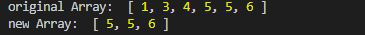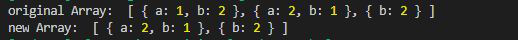# Lodash _.dropWhile() Method

• Last Updated : 29 Jul, 2020

Lodash is a JavaScript library that works on the top of underscore.js. Lodash helps in working with arrays, strings, objects, numbers etc.
The Loadsh.dropWhile() method is used to return the slice of the given array. This takes a predicate function that iterate through each element of the array and if the function returns false, it returned the sliced array excluding elements dropped from the beginning.

Syntax:

`dropWhile(array, [predicate=_.identity])`

Parameters:

• array: It is the array that is to be sliced.
• predicate: It is the function that either returns true or false depending on the condition given.

Return Value: It returns the new array after slicing.

Note: Please install lodash module by using command `npm install lodash` before using the code given below.

Example 1:

## Javascript

 `// Requiring the lodash library ` `const _ = require(``"lodash"``); ` ` `  `// Original array ` `let array1 = [1, 3, 4, 5, 5, 6] ` ` `  `// Using _.dropWhile() method ` `let newArray = _.dropWhile(array1, (e) => { ` `    ``return` `e != 5; ` `}); ` ` `  `// Original Array ` `console.log(``"original Array: "``, array1) ` ` `  `// Printing the newArray ` `console.log(``"new Array: "``, newArray) `

Output:Example 2: When array of object is given.

## Javascript

 `// Requiring the lodash library ` `const _ = require(``"lodash"``); ` ` `  `// Original array ` `let array1 = [ ` `    ``{ ``"a"``: 1, ``"b"``: 2 },  ` `    ``{ ``"a"``: 2, ``"b"``: 1 },  ` `    ``{ ``"b"``: 2 } ` `] ` ` `  `// Using _.dropWhile() method ` `let newArray = _.dropWhile(array1, (e) => { ` `    ``return` `e.b == 2; ` `}); ` ` `  `// Original Array ` `console.log(``"original Array: "``, array1) ` ` `  `// Printing the newArray ` `console.log(``"new Array: "``, newArray) `

Output:My Personal Notes arrow_drop_up
Recommended Articles
Page :